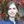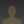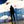0%
0%

# 7Wave Oscillator

A bench top oscillator which outputs seven different waveforms. Many waveform parameters are controllable.

Similar projects worth following
4.3k views
Originally intended as a VCO for a synthesizer, it turns out that voltage control is hard. So I'm making this one that could potentially be reconfigured as a VCO by changing out the frequency select stage with a voltage controlled resistor.

Output waveforms:
- Square
- Triangle (can be changed to ramp or saw, but changes the duty cycle of all the other waveforms)
- Ramp
- Saw
- Sine (can add an external integrator to produce cosine)
- Trapezoid
- PWM

Frequency range: With a switch that connects different capacitors in the inverting integrator, many different frequency ranges can be used. Components will be sized so that each capacitor covers about [0.8,1.2] of a decade (80 - 1200 Hz, for example). Target frequency range is 0.8 Hz - 12 kHz.

The switch in the Chopper will be a bjt.

So far, TL072 op amps are working well.

Tim Servo's circuits were very helpful in understanding waveshaping.

At this point, the most noteworthy part of this project is the spice model, shown to the left at the top. A lot of effort was expended to research, understand, and then fine tune each sub circuit.

The design is broken up into several sections. There's the triangle core, which produces the triangle and square waves, and then four wave shaping circuits which take the triangle and square waves and turn them into something else. There's also the LED drivers, which are connected to the triangle wave. They should show (at least at the lower frequencies) if the circuit is oscillating and how fast.

One part that isn't shown are the buffers. The amplitudes of each output waveform are different, and I want them to be 20 Vpp. Each buffer's gain will be tuned to give the desired amplitude.

I will post a project log as I breadboard each part.

### 7Wave - LTspice sim.asc

An LTspice simulation of the oscillator

asc - 14.69 kB - 02/22/2020 at 13:49

• ### Sine Shaping Circuit

[Work in progress. I may decrease the number of diode branches for simplicity.]

Progress has been made on the sine wave output. Apparently you can use a technique called diode wave shaping to turn a triangle wave into a sine wave. Here's the paper I found: http://tinyurl.com/lew34ez

Here's the schematic I went with:

Here's a useful graph:

And here's another:

In the first graph, each of the red x are the points in the wave when another diode stage is turned on. The slope of each line segment is directly related to the voltage division ratio.

In the second graph, I called the curve that my circuit is being designed to follow the shaping curve. I basically chopped a quarter cycle sine wave into four equal segments and linearized each segment.

I've started prototyping. The first thing to do is make what I'm calling the triangle core. It's the only circuit that's generating an independent waveform. Everything else is a type of wave shaping.

I made a breadboard layout (If anyone knows of a better program than PEBBLE to use, please inform):

And then I put it on the breadboard:

Here's a video of the triangle and square waves changing frequency and symmetry (duty cycle):

http://youtu.be/w_oxIqUgWuA

The triangle sides aren't straight. I suspect either the capacitor or weird diode things. Also, the frequency range isn't as wide as I would like it. I suspect the diode of dropping too much voltage.

In any case, further testing is required!

Share

## Discussions

zuul wrote 07/27/2014 at 02:02 point
cool

Are you sure? yes | no

## Similar Projects

Project Owner Contributor

### Musical Solid-State Tesla Coil v2Sebastian

Project Owner Contributor

### Open Source Bench Top Power Supplyacp.calin

Project Owner Contributor

### Palm-sized AC GeneratorEllie T

# Does this project spark your interest?

Become a member to follow this project and never miss any updates